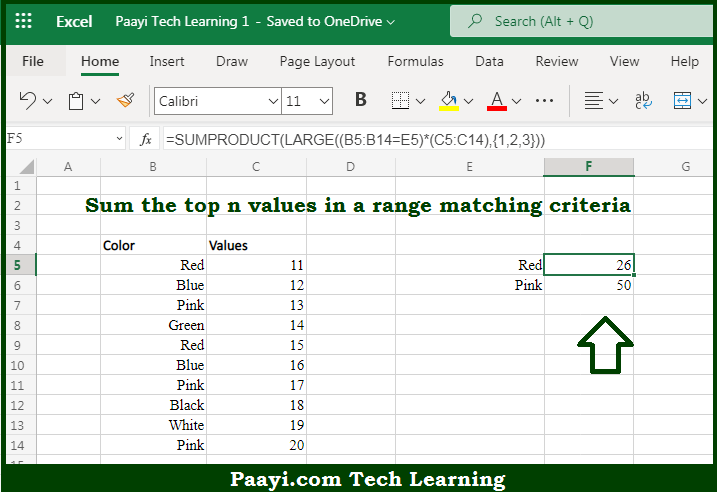# Learn How to SUM Top n Values With Criteria in Microsoft Excel

Written by | 0 Comments | 546 Views

In this article, you will learn how to SUM various things in Microsoft Excel using a single/combination(s) of functions. You will also know how to SUM Top n Values With Criteria and see the generic formula.

SUM Top n Values With Criteria in Microsoft Excel

The main purpose of this formula is to sum the top n values in a range matching criteria. Here we will learn how to sum the top n values with the provided criteria in the workbook in Microsoft Excel. That implies, with the help of a formula based on the LARGE and SUMPRODUCT function you can sum the top n values in a range matching criteria. So, with the help of this formula, you can able to sum the top n values with the provided criteria in the workbook in Microsoft Excel.

General Formula to SUM Top n Values

=SUMPRODUCT(LARGE((range=criteria)*(values),{1,2,3,N}))

The Explanation for the SUM Top n ValuesSo we know that with the help of the given formula above you can able to sum the top n values in a range matching criteria. Here we will learn how to sum the top n values with the provided criteria in the workbook in Microsoft Excel. As we know that the generic form of the formula provided here, rng represents a range of cells that contain numeric values and N represents the idea of Nth value. Besides that, the LARGE function will return the "Nth largest" value in a range. Also, we know that SUMPRODUCT is a flexible function that allows you to uses cell references for "k" inside the LARGE function. So, with the help of this formula, you can able to sum the top n values with the provided criteria in the workbook in Microsoft Excel.